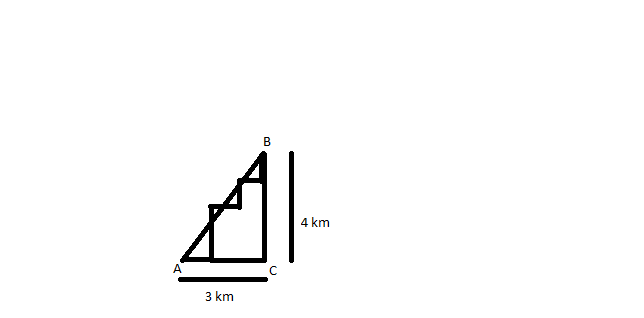Question 46

# A man starting at a point walks one km east, then two km north, then one km east, then one km north, then one km east and then one km north to arrive at the destination. What is the shortest distance from the starting point to the destination?

SolutionIn the diagram, A is starting point and B is ending point.
Accordingly, AB = $$\sqrt{(3^2 + 4^2)} = 5$$